# Three men

Alex is half younger than Jan, which is one-third younger than George. The sum of their ages is 48. How are these three men old?

a =  8
b =  16
c =  24

### Step-by-step explanation:

a = b - b/2
b = c - c/3
a+b+c= 48

2a-b = 0
3b-2c = 0
a+b+c = 48

a = 8
b = 16
c = 24

Our linear equations calculator calculates it.Did you find an error or inaccuracy? Feel free to write us. Thank you!Tips to related online calculators
Need help to calculate sum, simplify or multiply fractions? Try our fraction calculator.
Do you have a system of equations and looking for calculator system of linear equations?
Do you want to convert time units like minutes to seconds?

## Related math problems and questions:

• Happy marriageJan and Marienka have been living in a happy marriage for several years. Jan's age is written in the same numbers as Marienka only in reverse order. Their ages differ by just a fifth of Marienka's age. Jan is older. How old is Jan and how many are Marienk
• Two numbersWe have two numbers. Their sum is 140. One-fifth of the first number is equal to half the second number. Determine those unknown numbers.
• The sum 4The sum of Robin's age is 45. Seven years ago, Robin was 16 years more than one half as old as Bruno then. How old is Bruno?
• Three brothersThe three brothers have a total of 42 years. Jan is five years younger than Peter and Peter is 2 years younger than Michael. How many years has each of them?
• Jan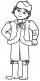Jan is 13 years and Peter 21 years old. For how many years will their ages in the ratio of 7:9?
• Two ages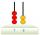Gabrielle is 9 years younger than Mikhail. The sum of their ages is 87. What is Mikhail's age?
• TheatroTheatrical performance was attended by 480 spectators. Women were in the audience 40 more than men and children 60 less than half of adult spectators. How many men, women, and children attended a theater performance?
• Two numbersThe sum of two numbers is 1. Identify this two numbers if you know that the half of first is equal to the third of second number.
• Age problemsA) Alex is 3 times as old as he was 2 years ago. How old is he now? b) Casey was twice as old as his sister 3 years ago. Now he is 5 years older than his sister. How old is Casey? c) Jessica is 4 years younger than Jennifer now. In 10 years, Jessica will
• Two numbersThe sum of the two numbers is 1. Find both numbers if you know that half of the first is equal to one seventh of the second number.
• Three friends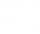Peter is eight times older than Rado, Rado is 3 times younger than Joseph. Together they have 120 years. Determine how many are each of them.
• The agesThe ages of the four sons make an arithmetic sequence, the sum of which is the age of the father today. In three years, the father's age will be the sum of the ages of the three eldest sons, and in the next two years and three months, the father's age wil
• EquationsSolve following system of equations: 6(x+7)+4(y-5)=12 2(x+y)-3(-2x+4y)=-44
• Mother and daughter 2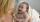The mother is 40 years older than her daughter. How old is the mother if her age is eight thirds age of daughter?
• Three siblings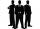Three siblings have birthday in one day-today. Together they have 35 years today. The youngest is three years younger than middle and the oldest is 5 years older than middle. How old is each?
• Saving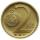Paul saves 155Kč in 46 2 Kc and 5Kc coins. How much saved 2Kc and 5Kc coins?
• Ages 2A man's age is 4 times his son's age. After 5 years he will be just twice his son's age, find their ages.